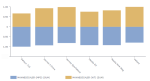# DCPY.MAXABSSCALER(column)

MaxAbsScaler scales data by dividing each value by largest absolute value in the column. It maps the absolute values to the range [0, 1]. When used on data with only positive values, it behaves like MinMaxScaler and also suffers from the presence of large outliers. Its main advantage is robustness against very small standard deviations.

###### Input data
• Numeric column
• Rows that contain missing values are dropped before calculations

Example: DCPY.MAXABSSCALER([Discount])

###### Result
• A numeric column with transformed values with the same length as the input column.
• Missing values are on the same indices like in the input column.
###### Key usage points
• Use it on data that does not contain large outliers, and is already centered at zero.
###### Example

The following example shows how the car weight and fuel economy (mpg) are scaled to the range from 0 to 1 by using the following functions:

• DCPY.MAXABSSCALER([MPG])

• DCPY.MAXABSSCALER([WT])

The two scaled values are visualized in the Butterfly visualization.For the whole list of algorithms, see Data science built-in algorithms.